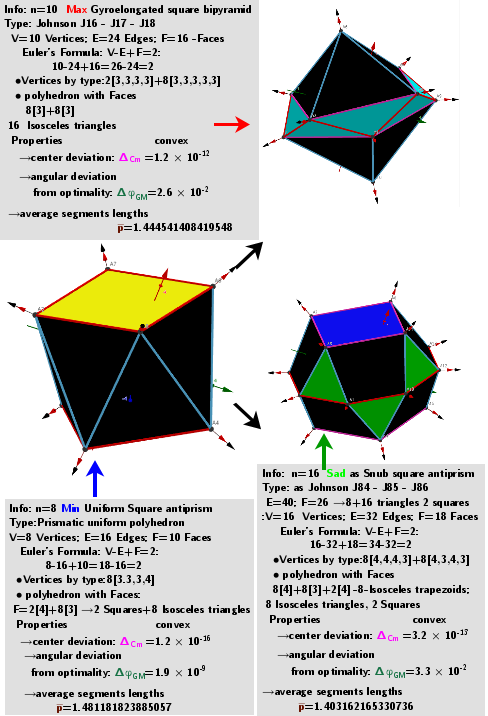# n=8 Square antiprism(anticube). Images: A critical points scheme for Generating uniformly distributed points on a sphereA system of points on a sphere S of radius R “induces” on the sphere S0 of radius R0 three different sets of points, which are geometric medians (GM) -local maxima, minima and saddle points sum of distance function f(x). The angular coordinates of the spherical distribution of a system of points - local minima coincide with the original system of points.